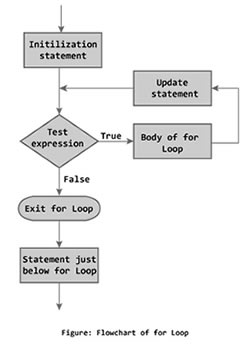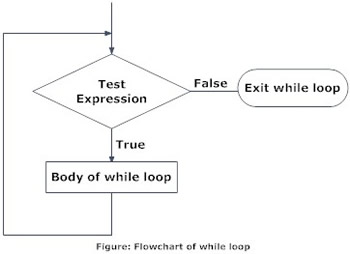# Difference between FOR and WHILE Loop With Example

##### Key Difference: The FOR loop is often used when you usually know how many times you would like the program, which means it will run that program until the number of times is complete before it terminates itself. The WHILE loop works in a similar manner but requires a conditional statement. Benefit of the WHILE loop is when you are unsure how many iterations are required to complete the given expression.Loops are often in programming and are useful if one needs to execute a program multiple times. These are often great when dealing with repetitive programming. These are often confusing for people who are just learning how to program.

While both FOR and WHILE loop are used for similar purpose of dealing with repetitive program until the conditions required are satisfied, they are used in different situations. Let’s understand each loop separately.

The FOR loop is often used when you usually know how many times you would like the program, which means it will run that program until the number of times is complete before it terminates itself. The FOR loop works by executing the initialization statement only once at the beginning of the loop. The test expression is then checked against the program. If the text expression is false, the loop is terminated; but if the expression is true then the code inside the body is executed and then update expression is updated. The loop continues until the text expression becomes false.

The FOR loop syntax looks something like this:

for(initialization statement; test expression; update statement) {

code/s to be executed;

}The WHILE loop works in a similar manner but requires a conditional statement. The WHILE loop checks if the test expression is true, based on which the loop will continue to terminate. If the condition inside the body of while loop is executed, that is, code/s inside the braces { } are executed. Then the updated loop is checked again against the test expression. The loop will continue to be executed until the test expression becomes false, at which point the loop will be terminated. Benefit of the WHILE loop is when you are unsure how many iterations are required to complete the given expression.

The WHILE loop syntax looks something like this:

while (test expression) {

statement/s to be executed.

}

Example of FOR loop provided by Programiz.com

#include <stdio.h>

int main(){

int n, count, sum=0;

printf("Enter the value of n.\n");

scanf("%d",&n);

for(count=1;count<=n;++count)  //for loop terminates if count>n

{

sum+=count;    /* this statement is equivalent to sum=sum+count */

}

printf("Sum=%d",sum);

return 0;

}

Example of WHILE loop provided by Programiz.com

/*C program to demonstrate the working of while loop*/

#include <stdio.h>

int main(){

int number,factorial;

printf("Enter a number.\n");

scanf("%d",&number);

factorial=1;

while (number>0){      /* while loop continues util test condition number>0 is true */

factorial=factorial*number;

--number;

}

printf("Factorial=%d",factorial);

return 0;

}

Comparison between FOR and WHILE Loop:

 FOR Loop WHILE Loop Definition To execute the certain block of code repeatedly until some conditions are satisfied Used for When you know the number of iterations beforehand When you have an idea about the range of values on which to iterate but are unsure of the exact number Loop Will loop until the number of mentioned iterations are completed Will loop until the condition presented is completed

Image Courtesy: programiz.com